# Simple angle meter using ADXL335 accelerometer [Arduino]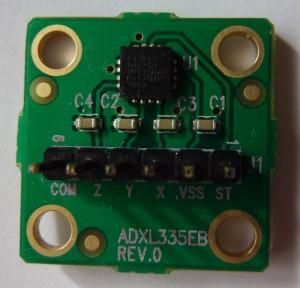ADXL335 is 3 axis accelerometer with analog output from Analog Devices. You can buy it as an evaluation kit  with standard 2,5 mm connector.

ADXL335 acceleration measurement range is +/- 3 g. Supply voltage is 1.8 –  3.6 V, however all specifications at the datasheet is given at 3.0 V. This accelerometer has  3 outputs for X,Y,Z axis which voltage is proportional to acceleration on specific axis.

At midpoint when acceleration is 0 g output is typically 1/2 of supply voltage. If a supply voltage is 3V, then output is 1.5 V. Output sensitivity typically is 300 mV/g.

The connecting ADXL335 to Arduino board is simple. The accelerometer can be supplied from the 3.3 V output at Arduino board, however then midpoint voltage and sensitivity is different from specified at datasheet. I used 3.0 V supply voltage, it came from voltage regulator LM317.

Outputs of X,Y,Z axis are connected directly to analog inputs of Arduino.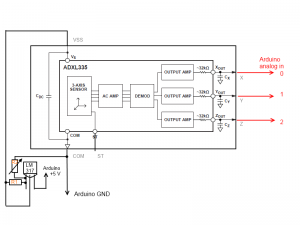R1 is 2.7k resistor, while R2 is variable 10k resistor. Please adjust R2 to have  3.0 V  output at LM317 before connecting ADXL335 board. Connect the board and adjust again, because output voltage will drop since load is connected. If  readings of the acceleration are unstable please connect additional capacitor between VSS and COM.

An accelerometer can be used not only measure acceleration, but also orientation. It’s because accelerometer sense the force of gravity which is pointed to the center of earth.

This is how sensing axis are orientated to ADXL335 (images from ADXL335 datasheet)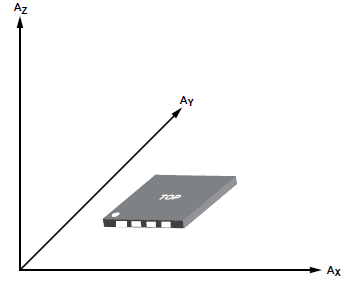Readings from X,Y,Z axis reflects an orientation of an accelerometer.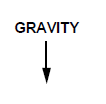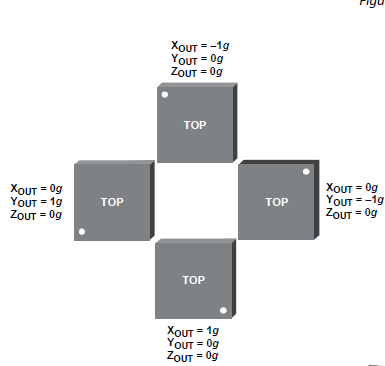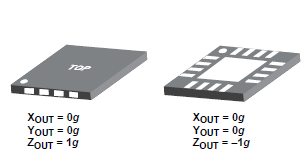Interesting that if a direction of sensing axis match to gravity direction measurement result is negative (-1 g).

Let’s start with an Arduino program. This program not only sends readings of acceleration from 3 axis via serial port, but also calculates rotation of the accelerometer around X, Y, Z, axis.

```

#define zero_x 1.569

#define zero_y 1.569

#define zero_z 1.569

#define sensitivity_x 0.3

#define sensitivity_y 0.3

#define sensitivity_z 0.3

```

ADC reference voltage is 2.56 V. Default “on board 5V” is not selected because is slightly lower then 5.0 V and can be unstable. 0 g voltage after some simple calibration is set to be 1.569 V. The sensitivity for calculations is used as default – 300 mV/g.

```void setup()   {

analogReference(INTERNAL2V56);

Serial.begin(256000);

}
```

Setting the ADC reference to 2.56 V and starting serial communication.

void loop() {

```

```

At the loop A/D conversions are performed for each of 3 channels.

```xv=(value_x/1024.0*ADC_ref-zero_x)/sensitivity_x;
```

The acceleration is calculated at this line.

```angle_z =atan2(-yv,-xv)*57.2957795+180;
```

The rotation(for Z axis) is calculated using atan2 function. It calculates angle from length of X, Y vectors. *57.2957795 – is conversation of radian to degree. +180 is for offset.

Full code:

```// Simple angle meter using ADXL335 accelerometer
//from electronicsblog.net/

#define zero_x 1.569

#define zero_y 1.569

#define zero_z 1.569

#define sensitivity_x 0.3

#define sensitivity_y 0.3

#define sensitivity_z 0.3

unsigned int value_x;
unsigned int value_y;
unsigned int value_z;

float xv;
float yv;
float zv;

float angle_x;
float angle_y;
float angle_z;

void setup()   {

analogReference(INTERNAL2V56);

Serial.begin(256000);

}

void loop() {

Serial.print ("x= ");
Serial.print (xv);
Serial.print(" g ");

Serial.print ("y= ");
Serial.print (yv);
Serial.print(" g ");

Serial.print ("z= ");
Serial.print (zv);
Serial.print(" g ");

Serial.print("\n");

Serial.print("Rotation ");

Serial.print("x= ");

angle_x =atan2(-yv,-zv)*57.2957795+180;

Serial.print(angle_x);
Serial.print(" deg");
Serial.print(" ");

Serial.print("y= ");

angle_y =atan2(-xv,-zv)*57.2957795+180;

Serial.print(angle_y);
Serial.print(" deg");
Serial.print(" ");

Serial.print("z= ");

angle_z =atan2(-yv,-xv)*57.2957795+180;

Serial.print(angle_z);
Serial.print(" deg");
Serial.print("\n");

delay(1000);
delay(1000);
}

```

And the demonstration of program in the video below.